Purva Huilgol — September 4, 2020

## Overview

• Precision and recall are two crucial yet misunderstood topics in machine learning
• We’ll discuss what precision and recall are, how they work, and their role in evaluating a machine learning model
• We’ll also gain an understanding of the Area Under the Curve (AUC) and Accuracy terms

## Introduction

Ask any machine learning professional or data scientist about the most confusing concepts in their learning journey. And invariably, the answer veers towards Precision and Recall.

The difference between Precision and Recall is actually easy to remember – but only once you’ve truly understood what each term stands for. But quite often, and I can attest to this, experts tend to offer half-baked explanations which confuse newcomers even more.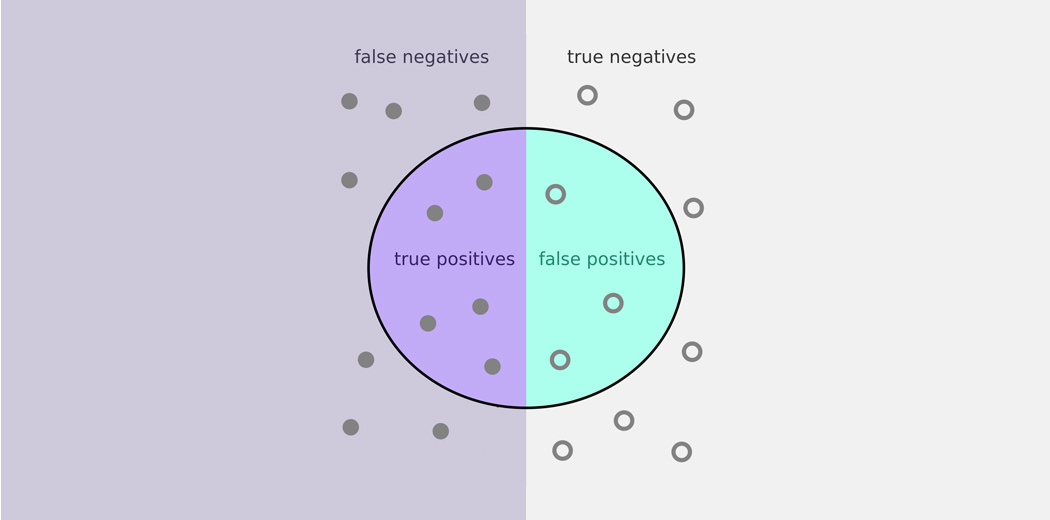For any machine learning model, we know that achieving a ‘good fit’ on the model is extremely crucial. This involves achieving the balance between underfitting and overfitting, or in other words, a tradeoff between bias and variance.

However, when it comes to classification – there is another tradeoff that is often overlooked in favor of the bias-variance tradeoff. This is the precision-recall tradeoff. Imbalanced classes occur commonly in datasets and when it comes to specific use cases, we would in fact like to give more importance to the precision and recall metrics, and also how to achieve the balance between them.

But, how to do so? We will explore the classification evaluation metrics by focussing on precision and recall in this article. We will also learn how to calculate these metrics in Python by taking a dataset and a simple classification algorithm. So, let’s get started!

You can learn about evaluation metrics in-depth here- Evaluation Metrics for Machine Learning Models.

1. Understanding the Problem Statement
2. What is Precision?
3. What is Recall?
4. The Easiest Evaluation Metric – Accuracy
5. The Role of the F1-Score
7. Understanding the Area Under the Curve (AUC)

## Understanding the Problem Statement

I strongly believe in learning by doing. So throughout this article, we’ll talk in practical terms – by using a dataset.

Let’s take up the popular Heart Disease Dataset available on the UCI repository. Here, we have to predict if the patient is suffering from a heart ailment or not using the given set of features. You can download the clean dataset from here.

Since this article solely focuses on model evaluation metrics, we will use the simplest classifier – the kNN classification model to make predictions.

As always, we shall start by importing the necessary libraries and packages:

Then let us get a look at the data and the target variables we are dealing with: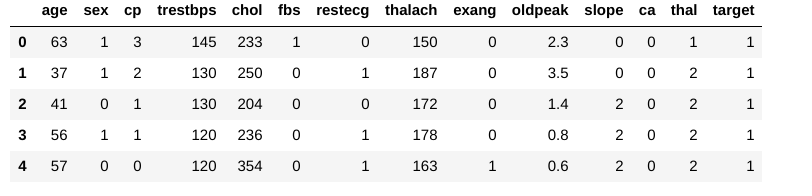Let us check if we have missing values: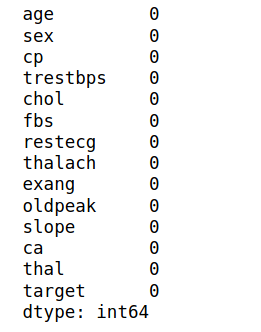There are no missing values. Now we can take a look at how many patients are actually suffering from heart disease (1) and how many are not (0):

This is the count plot below: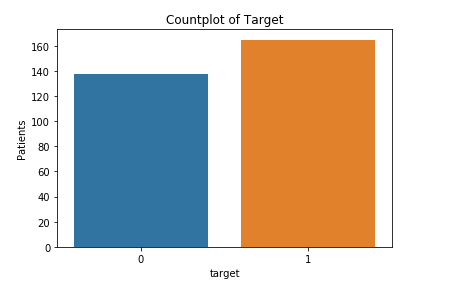Let us proceed by splitting our training and test data and our input and target variables. Since we are using KNN, it is mandatory to scale our datasets too:

The intuition behind choosing the best value of k is beyond the scope of this article, but we should know that we can determine the optimum value of k when we get the highest test score for that value. For that, we can evaluate the training and testing scores for up to 20 nearest neighbors:

To evaluate the max test score and the k values associated with it, run the following command: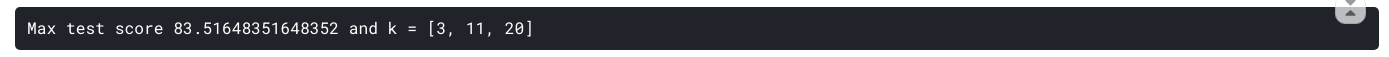Thus, we have obtained the optimum value of k to be 3, 11, or 20 with a score of 83.5. We will finalize one of these values and fit the model accordingly:Now, how do we evaluate whether this model is a ‘good’ model or not? For that, we use something called a Confusion Matrix: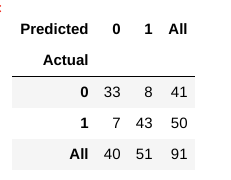A confusion matrix helps us gain an insight into how correct our predictions were and how they hold up against the actual values.

From our train and test data, we already know that our test data consisted of 91 data points. That is the 3rd row and 3rd column value at the end. We also notice that there are some actual and predicted values. The actual values are the number of data points that were originally categorized into 0 or 1. The predicted values are the number of data points our KNN model predicted as 0 or 1.

The actual values are:

• The patients who actually don’t have a heart disease = 41
• The patients who actually do have a heart disease = 50

The predicted values are:

• Number of patients who were predicted as not having a heart disease = 40
• Number of patients who were predicted as having a heart disease = 51

All the values we obtain above have a term. Let’s go over them one by one:

1. The cases in which the patients actually did not have heart disease and our model also predicted as not having it is called the True Negatives. For our matrix, True Negatives = 33.
2. The cases in which the patients actually have heart disease and our model also predicted as having it are called the True Positives. For our matrix, True Positives = 43
3. However, there are are some cases where the patient actually has no heart disease, but our model has predicted that they do. This kind of error is the Type I Error and we call the values as False Positives. For our matrix, False Positives = 8
4.  Similarly, there are are some cases where the patient actually has heart disease, but our model has predicted that he/she don’t. This kind of error is the Type II Error and we call the values as False Negatives.  For our matrix, False Negatives = 7

## What is Precision?

Right – so now we come to the crux of this article. What in the world is Precision? And what does all the above learning have to do with it?

In the simplest terms, Precision is the ratio between the True Positives and all the Positives. For our problem statement, that would be the measure of patients that we correctly identify having a heart disease out of all the patients actually having it. Mathematically: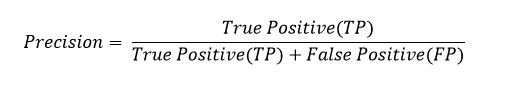What is the Precision for our model? Yes, it is 0.843 or, when it predicts that a patient has heart disease, it is correct around 84% of the time.

Precision also gives us a measure of the relevant data points. It is important that we don’t start treating a patient who actually doesn’t have a heart ailment, but our model predicted as having it.

## What is Recall?

The recall is the measure of our model correctly identifying True Positives. Thus, for all the patients who actually have heart disease, recall tells us how many we correctly identified as having a heart disease. Mathematically: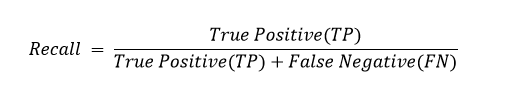For our model, Recall  = 0.86. Recall also gives a measure of how accurately our model is able to identify the relevant data. We refer to it as Sensitivity or True Positive Rate. What if a patient has heart disease, but there is no treatment given to him/her because our model predicted so? That is a situation we would like to avoid!

## The Easiest Metric to Understand – Accuracy

Now we come to one of the simplest metrics of all, Accuracy. Accuracy is the ratio of the total number of correct predictions and the total number of predictions. Can you guess what the formula for Accuracy will be?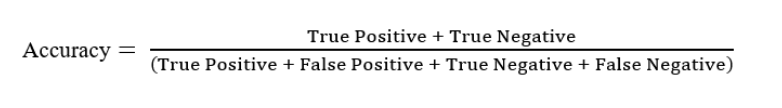For our model, Accuracy will be = 0.835.

Using accuracy as a defining metric for our model does make sense intuitively, but more often than not, it is always advisable to use Precision and Recall too. There might be other situations where our accuracy is very high, but our precision or recall is low. Ideally, for our model, we would like to completely avoid any situations where the patient has heart disease, but our model classifies as him not having it i.e., aim for high recall.

On the other hand, for the cases where the patient is not suffering from heart disease and our model predicts the opposite, we would also like to avoid treating a patient with no heart diseases(crucial when the input parameters could indicate a different ailment, but we end up treating him/her for a heart ailment).

Although we do aim for high precision and high recall value, achieving both at the same time is not possible. For example, if we change the model to one giving us a high recall, we might detect all the patients who actually have heart disease, but we might end up giving treatments to a lot of patients who don’t suffer from it.

Similarly, if we aim for high precision to avoid giving any wrong and unrequired treatment, we end up getting a lot of patients who actually have a heart disease going without any treatment.

## The Role of the F1-Score

Understanding Accuracy made us realize, we need a tradeoff between Precision and Recall. We first need to decide which is more important for our classification problem.

For example, for our dataset, we can consider that achieving a high recall is more important than getting a high precision – we would like to detect as many heart patients as possible. For some other models, like classifying whether a bank customer is a loan defaulter or not, it is desirable to have a high precision since the bank wouldn’t want to lose customers who were denied a loan based on the model’s prediction that they would be defaulters.

There are also a lot of situations where both precision and recall are equally important. For example, for our model, if the doctor informs us that the patients who were incorrectly classified as suffering from heart disease are equally important since they could be indicative of some other ailment, then we would aim for not only a high recall but a high precision as well.

In such cases, we use something called F1-score. F1-score is the Harmonic mean of the Precision and Recall: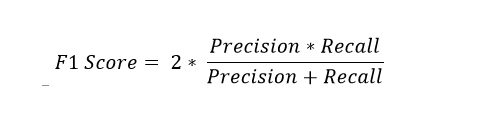This is easier to work with since now, instead of balancing precision and recall, we can just aim for a good F1-score and that would be indicative of a good Precision and a good Recall value as well.

We can generate the above metrics for our dataset using sklearn too: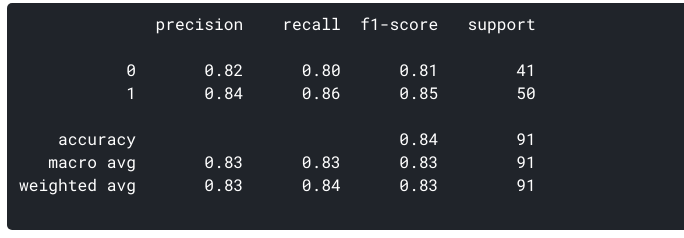## ROC Curve

Along with the above terms, there are more values we can calculate from the confusion matrix:

1. False Positive Rate (FPR): It is the ratio of the False Positives to the Actual number of Negatives. In the context of our model, it is a measure for how many cases did the model predicts that the patient has a heart disease from all the patients who actually didn’t have the heart disease.  For our data, the FPR is = 0.195
2. True Negative Rate (TNR) or the Specificity: It is the ratio of the True Negatives and the Actual Number of Negatives. For our model, it is the measure for how many cases did the model correctly predict that the patient does not have heart disease from all the patients who actually didn’t have heart disease. The TNR for the above data = 0.804. From these 2 definitions, we can also conclude that Specificity or TNR = 1 – FPR

We can also visualize Precision and Recall using ROC curves and PRC curves.

### 1. ROC Curves(Receiver Operating Characteristic Curve):

It is the plot between the TPR(y-axis) and FPR(x-axis). Since our model classifies the patient as having heart disease or not based on the probabilities generated for each class, we can decide the threshold of the probabilities as well.

For example, we want to set a threshold value of 0.4. This means that the model will classify the datapoint/patient as having heart disease if the probability of the patient having a heart disease is greater than 0.4. This will obviously give a high recall value and reduce the number of False Positives. Similarly, we can visualize how our model performs for different threshold values using the ROC curve.

Let us generate a ROC curve for our model with k = 3.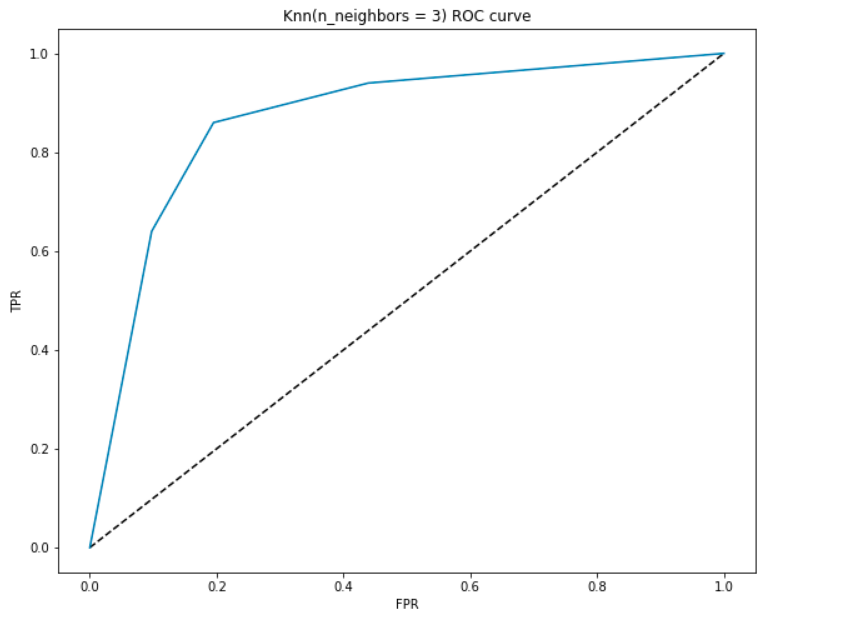#### AUC Interpretation-

• At the lowest point, i.e. at (0, 0)- the threshold is set at 1.0. This means our model classifies all patients as not having a heart disease.
• At the highest point i.e. at (1, 1), the threshold is set at 0.0. This means our model classifies all patients as having a heart disease.
• The rest of the curve is the values of FPR and TPR for the threshold values between 0 and 1. At some threshold value, we observe that for FPR close to 0, we are achieving a TPR of close to 1. This is when the model will predict the patients having heart disease almost perfectly.
• The area with the curve and the axes as the boundaries is called the Area Under Curve(AUC). It is this area which is considered as a metric of a good model. With this metric ranging from 0 to 1, we should aim for a high value of AUC. Models with a high AUC are called as models with good skill. Let us compute the AUC score of our model and the above plot:• We get a value of 0.868 as the AUC which is a pretty good score! In simplest terms, this means that the model will be able to distinguish the patients with heart disease and those who don’t 87% of the time. We can improve this score and I urge you try different hyperparameter values.
• The diagonal line is a random model with an AUC of 0.5, a model with no skill, which just the same as making a random prediction. Can you guess why?

### 2. Precision-Recall Curve (PRC)

As the name suggests, this curve is a direct representation of the precision(y-axis) and the recall(x-axis). If you observe our definitions and formulae for the Precision and Recall above, you will notice that at no point are we using the True Negatives(the actual number of people who don’t have heart disease).

This is particularly useful for the situations where we have an imbalanced dataset and the number of negatives is much larger than the positives(or when the number of patients having no heart disease is much larger than the patients having it). In such cases, our higher concern would be detecting the patients with heart disease as correctly as possible and would not need the TNR.

Like the ROC, we plot the precision and recall for different threshold values:

####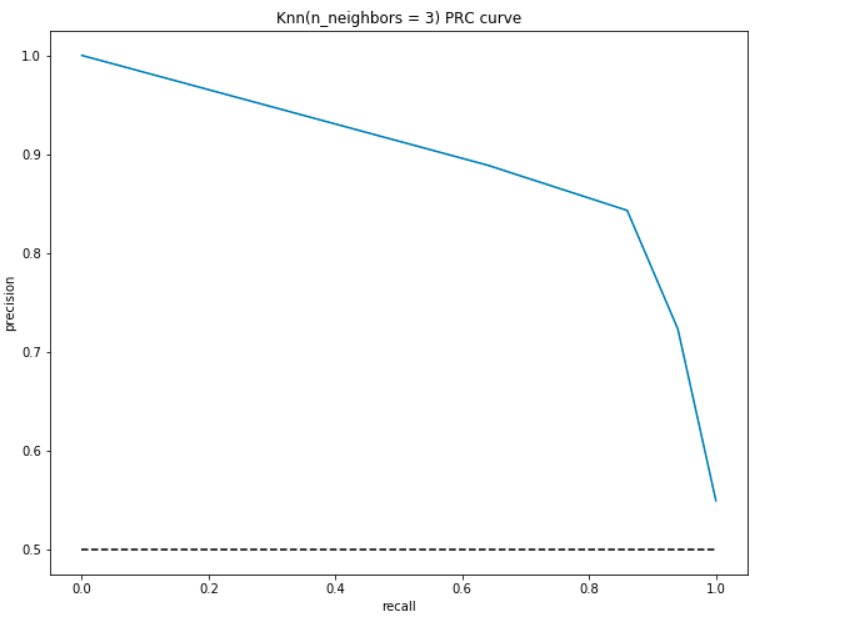PRC Interpretation:

• At the lowest point, i.e. at (0, 0)- the threshold is set at 1.0. This means our model makes no distinctions between the patients who have heart disease and the patients who don’t.
• At the highest point i.e. at (1, 1), the threshold is set at 0.0. This means that both our precision and recall are high and the model makes distinctions perfectly.
• The rest of the curve is the values of Precision and Recall for the threshold values between 0 and 1. Our aim is to make the curve as close to (1, 1) as possible- meaning a good precision and recall.
• Similar to ROC, the area with the curve and the axes as the boundaries is the Area Under Curve(AUC). Consider this area as a metric of a good model. The AUC ranges from 0 to 1. Therefore, we should aim for a high value of AUC. Let us compute the AUC for our model and the above plot:As before, we get a good AUC of around 90%.  Also, the model can achieve high precision with recall as 0 and would achieve a high recall by compromising the precision of 50%.

## End Notes

To conclude, in this article, we saw how to evaluate a classification model, especially focussing on precision and recall, and find a balance between them. Also, we explain how to represent our model performance using different metrics and a confusion matrix.

Here is an additional article for you to understand evaluation metrics- 11 Important Model Evaluation Metrics for Machine Learning Everyone should know

Also, in case you want to start learning Machine Learning, here are some free resources for you-###### Purva Huilgol